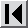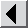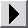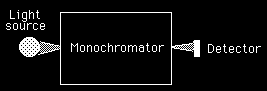## UV-Visible absorption spectrophotometer

UV-Visible absorption spectrophotometer with blackbody light source, grating monochromator, and photomultiplier detector. Assumptions: light source is imaged onto entrance slit; entrance and exit slits equal in size and shape; all light exiting the monochromator is detected by the PMT; stray light is unabsorbed by sample; absorption width is much greater than spectral bandpass. Limitations: no wavelength dependence of monochromator transmission factor (Top), stray light, and PMT sensitivity; no temperature dependence of PMT dark current.Variable sheet:

```---------------------------------------------------------------------------------------
St Input     Name    Output    Unit      Comment
freq    7.495E13  Hz        frequency (Hz)
E       4.966E-20 Joule     energy (Joule, erg, eV, kcal/mole)
v       2500      cm-1      wavenumber (cm-1)
400       lambda            nm        wavelength of light (mm, ü, Ąm, nm)
--------MONOCHROMATOR----------
1         m                           order (unitless)
d       .00083333 mm        grating constant (mm, cm, Ąm)
theta   14.386773 degrees   rotation angle of grating (radians, de
15        Phi               degrees   monochromator takeoff angle (radians,
alpha   29.386773 degrees   incidence angle (radians, degrees)
beta    .61322672 degrees   diffraction angle (radians, degrees)
1200      lines             lines/mm  grating ruling density (lines/mm)
AngDisp 1200.0687 mm-1      Angular dispersion (mm-1, nm-1)
LinDisp .36002062 mm/nm     Linear Dispersion (mm/mm, mm/nm, mm/ü)
RLD     2.7776187 nm/mm     Reciprocal linear dispersion (nm/mm, ü
300       Fm                mm        Focal length on monochromator (mm, cm,
Sg      1.3888093 nm        Geometrical spectral bandpass (mm, Ąm,
S       1.395476  nm        Spectral bandpass (mm, Ąm, nm, ü)
Snu     .8721725  cm-1      Spectral bandpass (cm-1)
5         H                 mm        Slit height (mm, Ąm)
.5        W                 mm        Slit width (mm, Ąm)
.5        Top                         Transmission factor of monochromator
Sd      .00666667 nm        diffraction limited resolution (mm, Ąm
N       60000               Total number of lines in grating (unit
50        Wg                mm        aperture (mm, cm, m)
omega   .02420353 sr        acceptance solid angle, steradians
Fnumber 5.6964669           F-number of monochromator
As      .025      cm2       Slit area (cm2, mm2)
Ap      21.783178 cm2       grating area viewed from entrance slit
PhiIn   .02132818 watts     Radiant power input into monochromator
.01       Strayli                     Percent stray light
Phi0    1.3896E-7 watts     Radiant power passed by monochromator
---------LIGHT SOURCE-------------
.5        emisiv                      emissivity of blackbody
2500      Tb                K         Temperature of blackbody (K, C)
nm      400       nm        wavelength in nm
peak    1158.8    nm        Peak wavelength  (nm, ü, Ąm, cm)
B       35.248047 W/sr/cm2  Total radiance over all wavelengths
Blambda .00033073 W/sr/nm/c Source spectral radiance (W/sr/nm/cm2)
-----PHOTOMULTIPLIER DETECTOR---------
.2        Klam                        Cathode quantum efficiency
g       3.2150301           gain per stage
SEF     .45146114           secondary emission factor
600       V                 volts     total voltage applied
Vd      66.666667 volts     voltage per dynode (volts, KV)
9         k                           number of dynodes
M       36699.941           multiplication factor
1000      rt                sec-1     cathode thermionic emission rate (sec-
.9        eta                         collection efficiency
Rlam    .64515324 amps/watt radiant cathode responsivity (amps/wat
Flux    2.7981E12 sec-1     Photon flux on cathode (sec-1)
rcp     5.5963E11 sec-1     cathode photoelectron emission rate, s
ic      8.9652E-8 amps      cathode photocurrent (amps, mA, ĄA, nA
ict     1.602E-16 amps      cathode thermionic dark current (amps,
iat     5.291E-12 amps      anode thermionic dark current (amps, m
303       T                 K         Temperature of load resistor (K, C)
1000      RL                ohms      Load (or feedback) resistance (ohms, K
sigmai  5.0269E-9 amps      signal shot noise current (amps, mA, Ą
sigmat  2.125E-13 amps      thermionic shot noise current (amps, m
0         excess            amps      excess noise current (amps, mA, ĄA, nA
sigmad  2.125E-13 amps      total dark noise current (amps, mA, ĄA
sigma   5.0269E-9 amps      total shot noise current (amps, mA, ĄA
sigmav  5.0269E-6 volts     total shot noise voltage (volts, mvolt
sigmaJ  2.8919E-9 volts     Johnson noise voltage (volts, mvolts)
0         flicfac                     flicker factor
sigmaf  0         volts     flicker noise voltage (volts, mvolts)
sigman  5.0269E-6 volts     total noise voltage (volts, mvolts)
1         t                 sec       integration time (sec)
DeltaF  .5        Hz        noise bandwidth, Hz
tau     .5        sec       RC time constant (sec)
resptim 2.5       sec       RC response time (sec)
ia      .00296121 amps      reference photocurrent  (amps, mA, ĄA,
L            SNR     589130.53           signal-to-noise ratio of incident inte
----SAMPLE and OUTPUT SIGNAL-------
1         a                           absorptivity of analyte
1         b                 cm        path length of cell
1         c                           concentration of analyte
A       1                   true aborbance
ias     .00029642 amps      sample photocurrent  (amps, mA, ĄA, nA
Es      2.9615091 volts     reference signal voltage (volts, mvolt
Ess     .29641742 volts     sample signal voltage (volts, mvolts)
sigmas  1.5904E-9           sample shot noise current (amps, mA, Ą
Aobs    .99960934           Measured absorbance
RSDref  1.6976E-6           RSD of reference signal
RSDsamp 5.3656E-6           RSD of sample signal
RSD     5.6277E-6           RSD of transmission
RSDA    .00024434           Percent RSD of absorbance
---------------------------------------------------------------------------------------

Rule sheet:
---------------------------------------------------------------------------------------
RSDA=100*.434*RSD/Aobs  d=1/lines
AngDisp = m/(d*cos(beta))
LinDisp = Fm*AngDisp
RLD=1/LinDisp
Sg=W/LinDisp
S = Sg+Sd
Snu=.1*S/(lambda*lambda)
Sd = lambda/(m*N)
N = Wg/d
beta=Phi-theta
alpha=theta+Phi
m*lambda=2*d*cos(Phi)*sin(theta)
omega=Ap/Fm^2
Ap=Wg^2*cos(alpha)
PhiIn=B*As*omega
As=0.01*W*H
Phi0=Blambda*As*omega*Top*Sg*1e6
Fnumber=Fm/sqrt(4*Ap/pi())
lambda = 1/v
Blambda = emisiv*1.19111E16*nm^-5/(exp(1.438e7/(nm*Tb))-1)
E = (6.6261E-34)*freq
freq = (2.998E10)/lambda
B=emisiv*1.8047e-12*Tb^4
peak=2.897e6/Tb
nm=lambda*1e6
exponent=1.438e7/(nm*Tb)
LambdaT=nm*Tb
lambda = 1/v
E = (6.6261E-34)*freq
freq = (2.998E10)/lambda
M=g^k
Vd=V/k
g=0.17*Vd^.7    " this is partly empirical
rcp=Klam*Flux
Rlam=(Klam*1.602e-19)/E
ia=eta*M*Rlam*Phi0
ic=rcp*1.602e-19
Flux=Phi0/E
ict=rt*1.602e-19
iat=M*eta*ict
Es=RL*((ia*Straylight/100)+ia+iat)
sigmai=sqrt(2*1.602e-19*(1+SEF)*M*ia*DeltaF)
SEF=1/(g-1)
sigmat=sqrt(2*1.602e-19*(1+SEF)*M*iat*DeltaF)
sigmaJ=sqrt(4*1.38e-23*T*RL*DeltaF)
sigman=sqrt((RL*sigma)^2+sigmaJ^2+sigmaf^2)
sigmav=RL*sigma
SNR=Es/sigman
DeltaF=1/(2*t)
DeltaF=1/(4*tau)
resptime=5*tau
sigmaf=flicfac*Es
A=a*b*c
ias=(ia*Straylight/100)+ia*10^(-A)
Ess=RL*(ias+iat)
sigmas=sqrt(2*1.602e-19*(1+SEF)*M*(ias+iat)*DeltaF)
Aobs=log(Es/Ess)
RSDref=sigmai/ia
RSDsamp=sigmas/ias
RSD=sqrt(RSDref^2+RSDsamp^2)
RSDA=100*.434*RSD/Aobs
---------------------------------------------------------------------------------------```
You may download the UV-Visible absorption spectrophotometer model in either binary or BIHHEX (text-encoded) mode.